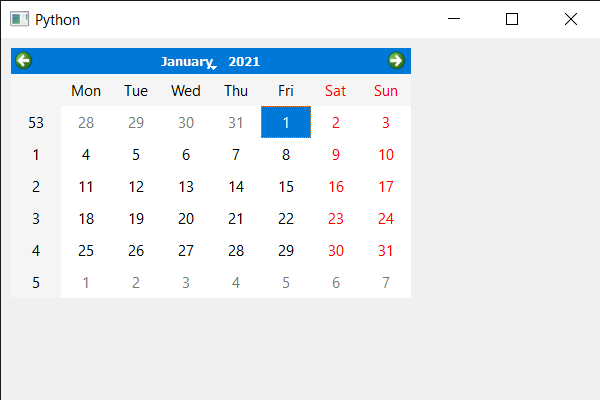Open in App
Not now

# PyQt5 QCalendarWidget – Setting Selected Date

• Last Updated : 04 Jul, 2021

In this article we will see how we can set the selected date of QCalendarWidget, selected date is the date that is currently highlighted user can change the selected date using the mouse and cursor by default selected date is the real-time date although we can set the selected date any time.
Note: The selected date must be within the date range specified by the minimumDate and maximumDate properties.

In order to do this we will use setSelectedDate method with the QCalendarWidget object.
Syntax : calendar.setSelectedDate(date)
Argument : It takes QDate object as argument
Return : It returns None

Below is the implementation

## Python3

 `# importing libraries``from` `PyQt5.QtWidgets ``import` `*``from` `PyQt5 ``import` `QtCore, QtGui``from` `PyQt5.QtGui ``import` `*``from` `PyQt5.QtCore ``import` `*``import` `sys`  `class` `Window(QMainWindow):` `    ``def` `__init__(``self``):``        ``super``().__init__()` `        ``# setting title``        ``self``.setWindowTitle(``"Python "``)` `        ``# setting geometry``        ``self``.setGeometry(``100``, ``100``, ``600``, ``400``)` `        ``# calling method``        ``self``.UiComponents()` `        ``# showing all the widgets``        ``self``.show()` `    ``# method for components``    ``def` `UiComponents(``self``):` `        ``# creating a QCalendarWidget object``        ``calendar ``=` `QCalendarWidget(``self``)` `        ``# setting geometry to the calendar``        ``calendar.setGeometry(``10``, ``10``, ``400``, ``250``)` `        ``# date``        ``date ``=` `QDate(``2021``, ``1``, ``1``)` `        ``# setting selected date``        ``calendar.setSelectedDate(date)`  `# create pyqt5 app``App ``=` `QApplication(sys.argv)` `# create the instance of our Window``window ``=` `Window()` `# start the app``sys.exit(App.``exec``())`

Output :My Personal Notes arrow_drop_up